# AP Statistics : How to test of significance for the slope of a least-squares regression line

## Example Questions

### Example Question #1 : Mean And Linear Regression

A statistician conducts a regression analysis and obtains a p-value of 0.1. It is more likely than not that there is a relationship between the variables in the study.

False

True

True

Explanation:

A p-value of 0.1 is generally not sufficient to reject the null hypothesis, but this is only because we want a high degree of confidence before finding a relationship between variables.  Here, there is most likely a relationship between the variables even though the statistician could not reject the null hypothesis.

### Example Question #2 : Mean And Linear Regression

For a data set, the least-squares regression line has a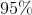confidence interval for the slope of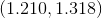.

Based on this confidence interval, what can you do with a hypothesis test at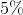significance level where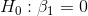and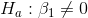?

Fail to reject the null hypothesis because this confidence interval does not include.

Fail to reject the null hypothesis because this confidence interval does not include.

Not enough information to be able to decide.

Reject the null hypothesis because this confidence interval does not include.

Reject the null hypothesis because this confidence interval does not include.

Reject the null hypothesis because this confidence interval does not include.

Explanation:

Notice that the interval does not include. This means that the P-value for the hypothesis test would be under 5%, which would lead us to reject our null hypothesis.

Any confidence interval can be used to create a hypothesis test by inverting it, and it is fairly simple, but the concept is tested into graduate-level statistics theory.

### Example Question #3 : Mean And Linear Regression

Which of the following is an incorrect condition requirement for regression inference?

A trend/pattern of some sort in the residual plot

The standard deviation of the response must be constan

Ordered pairs must be independent of each other

A linear relationship betweenandResponse must vary normally about the regression line for any given value of